Paired T-test Walkthrough

Part I: Introduction

This script walks through a paired t-test and other basic data-investigation tasks in 'R'.

Data collection

The data come from an experiment where the effects of a parasitic insects were assessed on Solidago candensis plants. Plants with rosette galls (Figure 1) were haphazardly chosen in an abandoned agricultural field near the University of Pittsburgh Pymatuning Laboratory of Ecology in north-west Pennsylvania, USA. An adjacent plant that was not galled was selected as a paired control. Plant height was measured in the field and the plants were cut, dried and massed. The hypothesis is that galled plants should on average have fewere resources to allocate to growth and reproduction. Data was collected by Pitt Biological Sciences students taking Dr. Walt Carson's Ecology Laboratory class.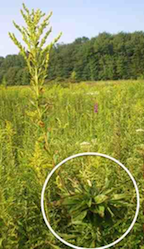Fig 1: Rosette Gall on S. canadensis

This script is design to be a walk through of some basic 'R' tasks. This experiment is conducted each year for the Ecology Lab so this script is also design to automate analyses of new datasets to facilitate grading. There are therefore multiple t-tests that are fairly similar to each other in terms of purpose and code at the end.

The main tasks of this exercise are:

• Load data from a dataframe
• Clean & set up data
• Create new data columns
• Investigate summary stats
• Conduct paired t-tests
• Create bar plots

Functions used:

Functions used in the script include: 'remove', 'getwd', 'setwd', 'list.files', 'dim', 'names', 'head', 'is', 'summary', 'factor', 'attach', 'any', 'cbind', 'ifelse', 'write.csv', 'save.image', 'read', 't.test', 'boxplot'

Preliminaries

Clear out the existing workspace. This removes any and all objects and data that you've worked with since you opend 'R'.

remove(list = ls())

Set working directory for where data is located

# My current working directory ('wd')
getwd()
##  "/Users/nathanbrouwer/R/Golden_Rod"

# Set the working directory for where data is located
setwd("~/R/Golden_Rod")

Take look at the files in your current working directory. If I issued the command 'list.files()' it would show everything in the working directory. Since I've included 'pattern = ' I just get files that contain the word “GoldenRod”.

list.files(pattern = "GoldenRod")
##  "GoldenRod_2012_ORIG.xls"   "GoldenRod_2013_EDIT_1.csv"
##  "GoldenRod_2013_EDIT_1.xls" "GoldenRod_2013_EDIT_2.csv"
##  "GoldenRod_2013_ORIG.xls"

Load and examine the structure of the data

Load data into an object called “data”

Look at the structure of the data in the object called “data”

How big is the dataframe?

dim(data)
##  70  8

What are the names of the columns in it?

names(data)
##  "pair"        "gall_YN"     "height"      "weight_STEM" "weight_FLWR"
##  "X"           "X.1"         "X.2"

Now look at the first few rows of data

##   pair gall_YN height weight_STEM weight_FLWR  X X.1 X.2
## 1    1       N  102.0         9.7         1.0 NA  NA  NA
## 2    2       N   84.1         8.6         0.6 NA  NA  NA
## 3    3       N   76.4         9.0         1.1 NA  NA  NA
## 4    4       N   98.6         9.4         1.8 NA  NA  NA
## 5    5       N  116.9         8.3         0.7 NA  NA  NA
## 6    6       N  105.7        15.7         3.1 NA  NA  NA

Notice that there are 3 columns with weird names and no data. There are artifacts from the the original Excel sheet. I am going to re-open, delete the 3 columns to the right of the “weight_FLWR” column, and save the .csv fileas a new file name “GoldenRod_2013_EDIT_2.csv”. They will probabably appear to be empty but for some reason there is some residual Excel stuff in them. This is a common occurrence.

Reload data, overwriting original “data” object

data <- read.csv("GoldenRod_2013_EDIT_2.csv", header = TRUE)  #note that I've updated the file name to 'edit_2'

There are only 5 columns here

dim(data)
##  70  5

The “data” object I've created is in the format of a “dataframe” For preliminary R work you will generally only use dataframes. If forsome reason you need to know what format an object is you can use the is() command

is(data)
##  "data.frame" "list"       "oldClass"   "vector"

If I isolated just a single column of the data and save it to an object, it will be in a “vector” format.

data.weight_STEM <- data\$weight_STEM

# The indication that the object is a vector is the second item in the list
# of information.
is(data.weight_STEM)
##  "numeric" "vector"

Summary info on data

One of the most useful commands in R is summary(). summary() lists the 5 columns of data and relevant summary inform for each column. Based on the output, you can infer what type of statistical variable is in each column. Numeric variables have means and other information report. For categorical variables (aka “factor” variables) you see the of individuals in each category.

summary(data)
##       pair       gall_YN     height       weight_STEM     weight_FLWR
##  Min.   : 1.00   N:35    Min.   : 76.4   Min.   : 5.30   Min.   :-1.100
##  1st Qu.: 9.25   Y:35    1st Qu.: 90.8   1st Qu.: 9.25   1st Qu.: 0.300
##  Median :18.00           Median :104.5   Median :11.70   Median : 0.650
##  Mean   :18.00           Mean   :104.7   Mean   :12.00   Mean   : 0.964
##  3rd Qu.:26.75           3rd Qu.:113.5   3rd Qu.:14.38   3rd Qu.: 1.675
##  Max.   :35.00           Max.   :146.2   Max.   :20.00   Max.   : 4.500

In this Solidago data set these columns mean:

• pair = categorical variable designating which pairs of plants; one plant had a gall, the other not
• gall_YN = cateorical variable designating whether plant was parasitized and has a rosette gall. Specifically, its a binary cateogrical variable.
• height = height of the plant stem in cm
• weight_STEM = mass of the stem, leaves, and the rosette gall, if plant was galled
• weight_FLWR = mass in grams of the plants flower, if the plant had one.

Changing data types of columns

The command we used to read-in the data, read.csv(), attempts to detect what each column is. Usually it does a pretty good job, though it is very sensitive to typos and has some other idiosyncracies. Working with factor variables/ categorical variables can be one of the more frustrating aspects of R. In this case, however, everything looks good except that above we said that “pair” was a categorical variable and the summary() comman is treating it as numeric. This is apparent because it has a mean, median, etc, while for the correctly categorized categorical variable, gall_YN we just see a count of the number of each each category..

The pair column was assigned to being a numeric variable because it uses numbers in the original excel file, so the read.table command assumed it was numeric. If the pairs had been given letters a, b, c etc R would have correctly designated it a cateogrical variable.

We can easily fix this using the “factor()” command. This is exactly the same as the “as.factor()” command (as.factor is the same as factor).

Change pair to factor

data\$pair <- factor(data\$pair)

The arrow looking thing “<-” is called an assignment. So, we are re-assigning the column of information data\$pair as a factor. Let's see how this changes the output of the summary command

summary(data)
##       pair    gall_YN     height       weight_STEM     weight_FLWR
##  1      : 2   N:35    Min.   : 76.4   Min.   : 5.30   Min.   :-1.100
##  2      : 2   Y:35    1st Qu.: 90.8   1st Qu.: 9.25   1st Qu.: 0.300
##  3      : 2           Median :104.5   Median :11.70   Median : 0.650
##  4      : 2           Mean   :104.7   Mean   :12.00   Mean   : 0.964
##  5      : 2           3rd Qu.:113.5   3rd Qu.:14.38   3rd Qu.: 1.675
##  6      : 2           Max.   :146.2   Max.   :20.00   Max.   : 4.500
##  (Other):58

R now treats pair as a factor. Note that it only shows the first six “levels” of this factor, which in this case are just the ID numbers 1 thru 6. There are two rows of data at each level because in this experiment one member of a pair is galled and the other not galled.

If you have a factor with more than 6 levels you can show more of them by adding “maxsum” to the call to summary.

summary(data, maxsum = 10)
##       pair    gall_YN     height       weight_STEM     weight_FLWR
##  1      : 2   N:35    Min.   : 76.4   Min.   : 5.30   Min.   :-1.100
##  2      : 2   Y:35    1st Qu.: 90.8   1st Qu.: 9.25   1st Qu.: 0.300
##  3      : 2           Median :104.5   Median :11.70   Median : 0.650
##  4      : 2           Mean   :104.7   Mean   :12.00   Mean   : 0.964
##  5      : 2           3rd Qu.:113.5   3rd Qu.:14.38   3rd Qu.: 1.675
##  6      : 2           Max.   :146.2   Max.   :20.00   Max.   : 4.500
##  7      : 2
##  8      : 2
##  9      : 2
##  (Other):52

The “\$” character is used to access a column within a dataframe object. So the code “data\$pair” means “the pair column within the data object”. We can get summary information on just the pair column like this

summary(data\$pair)
##  1  2  3  4  5  6  7  8  9 10 11 12 13 14 15 16 17 18 19 20 21 22 23 24 25
##  2  2  2  2  2  2  2  2  2  2  2  2  2  2  2  2  2  2  2  2  2  2  2  2  2
## 26 27 28 29 30 31 32 33 34 35
##  2  2  2  2  2  2  2  2  2  2

This shows us that there are 35 levels to the pair factor, named 1 thru 35, and that there are two rows of data assigned to each level of the pair factor

What happens if we leave out the summary command?

data\$pair
##   1  2  3  4  5  6  7  8  9  10 11 12 13 14 15 16 17 18 19 20 21 22 23
##  24 25 26 27 28 29 30 31 32 33 34 35 1  2  3  4  5  6  7  8  9  10 11
##  12 13 14 15 16 17 18 19 20 21 22 23 24 25 26 27 28 29 30 31 32 33 34
##  35
## 35 Levels: 1 2 3 4 5 6 7 8 9 10 11 12 13 14 15 16 17 18 19 20 21 22 ... 35

We get the raw data. We also get a little bit of information about it at the bottom of the output where it says “35 levels…” indcating that this is a categorical variable

If it was a call to the gall_YN column we get similar information

data\$gall_YN
##   N N N N N N N N N N N N N N N N N N N N N N N N N N N N N N N N N N N
##  Y Y Y Y Y Y Y Y Y Y Y Y Y Y Y Y Y Y Y Y Y Y Y Y Y Y Y Y Y Y Y Y Y Y Y
## Levels: N Y

What if we just type in gall_YN?

gall_YN

Nothing. gall_YN is not an object. However, something people often do, especially when doing basic R teaching, is use the attach command. This tells R to recognize the columns within the data object as objects thesmelves

attach(data)

Now we get something different if we call up one of the columns from data directly

gall_YN
##   N N N N N N N N N N N N N N N N N N N N N N N N N N N N N N N N N N N
##  Y Y Y Y Y Y Y Y Y Y Y Y Y Y Y Y Y Y Y Y Y Y Y Y Y Y Y Y Y Y Y Y Y Y Y
## Levels: N Y

This information is dirrectly equivalent to data\$gall_YN. We can ask R to confirm this like this.

gall_YN == data\$gall_YN
##   TRUE TRUE TRUE TRUE TRUE TRUE TRUE TRUE TRUE TRUE TRUE TRUE TRUE TRUE
##  TRUE TRUE TRUE TRUE TRUE TRUE TRUE TRUE TRUE TRUE TRUE TRUE TRUE TRUE
##  TRUE TRUE TRUE TRUE TRUE TRUE TRUE TRUE TRUE TRUE TRUE TRUE TRUE TRUE
##  TRUE TRUE TRUE TRUE TRUE TRUE TRUE TRUE TRUE TRUE TRUE TRUE TRUE TRUE
##  TRUE TRUE TRUE TRUE TRUE TRUE TRUE TRUE TRUE TRUE TRUE TRUE TRUE TRUE

Now for a touch of fancy code to an otherwise trivial example. We can ask R if any of the elements in the command gall_YN == data\$gall_YN equal False, which would mean there was some sort of mismatch. This can be done with the any command.

any(c(gall_YN == data\$gall_YN) == "FALSE")
##  FALSE

Cacluate new data columns

For our anlaysis we'll want to look not only at the mass of the plants, but all the relative allocation to flowering vs. stems. We can easily have R do the math and add new columns to the dataframe for us.

In order to get the total mass of each plant we'll add together the weight of the stem and the weight of the flower. I'll show two ways to do this. One is the fast, 1-step way, the other slow but explicit way.

# 1st, the fast way: create new data and add to dataframe in one step
data\$weight_TOTAL <- data\$weight_STEM + data\$weight_FLWR

# 2nd, the slow way.  Create new data and save it as an object
weight_TOTAL <- data\$weight_STEM + data\$weight_FLWR

# Add that object to existing dataframe
data <- cbind(data, weight_TOTAL)

Method 2 is perfectly ok, but takes more code and makes an extra object within your workspace. Some people prefer this way because it lays out everythings step by step. Note that right now I have two identical. weight_TOTAL columns.

names(data)
##  "pair"         "gall_YN"      "height"       "weight_STEM"
##  "weight_FLWR"  "weight_TOTAL" "weight_TOTAL"

This won't cause a problem, unless for some reason the data in the two identical columns are different. Then I'd need to get rid of one. One way to do this is to re-run all of the code and skip the step that the made two identical columns. This is what I used to do and can be annoying. The easiest way to do this task is to use a convention of R called “negative indexing”. I can see that the identical columns are in columns 6 and 7. I can access any column in a dataframe, or multiple columns, using square brackets. So, if I want to see column 7, one of my identical columns, I call this

data[, 7]
##   10.7  9.2 10.1 11.2  9.0 18.8 11.7 10.2  7.6 19.0 12.3 14.9 13.0 12.0
##  13.7 15.4 10.6 14.4  9.9 10.7 16.1 22.1  8.7 18.3 18.4 13.7  5.6  8.5
##  13.4 14.7 19.8 14.4 17.0 14.2 17.8  8.1  7.2  9.4  8.0 10.3 19.9 11.7
##  12.6  9.7 14.4 12.3 12.8 18.6  8.7 11.7 10.9 10.3 14.6  8.5 10.0 19.9
##  19.5 10.0  7.7 21.7 10.7 15.5 13.7  9.3 11.2 18.9 16.9 11.7 13.4 10.7

To remove columns 7 I can apply negative indexing

# Remove the column
data <- data[, -7]

# Check the modified data
names(data)
##  "pair"         "gall_YN"      "height"       "weight_STEM"
##  "weight_FLWR"  "weight_TOTAL"

Negative indexing is a more advanced approach to manipulating data and might not be the first thing that comes to mind when you have to deal with something like a duplicated column. Another approach, and a good practice in general, is to rename your dataframe as you make modifications to it. I'll do this for our next data column, the percentage of each plant's mass that occurred in flowers.

# Make a new object
data.v2 <- data

data.v2\$percent_FLWR <- data.v2\$weight_FLWR/data.v2\$weight_TOTAL

One drawback of this approach is you can end up with a proliferation of different versions of the data frame, and it can be easy to make a typo that leaves of part of the name and is hard to notice. One approach I use to keep things more manageable is to make a “backup” and “working” version of the dataframe.

data.backup <- data.v2

data.working <- data.backup

To be extra-safe I can save the data as a .csv file. Just as I used read.csv to get data in to R from a spreadsheet, I used write.csv to get it out. I just feed write.csv the object I want to save in spread-sheet format and a file name in quotes.

write.csv(data.backup, file = "Solidago_processed_vs3.csv")

Saving to a CSV file is nice because it backup your work and there's no way to change the file from within your R session, unless you accidentally save over it. I can re-load this data if I closed my sessions or made a mistake.

# Check it out
##   X pair gall_YN height weight_STEM weight_FLWR weight_TOTAL percent_FLWR
## 1 1    1       N  102.0         9.7         1.0         10.7      0.09346
## 2 2    2       N   84.1         8.6         0.6          9.2      0.06522
## 3 3    3       N   76.4         9.0         1.1         10.1      0.10891
## 4 4    4       N   98.6         9.4         1.8         11.2      0.16071
## 5 5    5       N  116.9         8.3         0.7          9.0      0.07778
## 6 6    6       N  105.7        15.7         3.1         18.8      0.16489

One thing you'll notice is that there is now a new column X, on the left-hand side of the dataframe. This was put there by write.csv as an index. This servers no purpse and, if I were to now save my new object data.again to a csv file, it would end up with two “X” columns. To get around this you add row.names = FALSE to the command.

# Save it again, intentionally overwriting the 1st file
write.csv(data.backup, file = "Solidago_processed_vs3.csv", row.names = FALSE)

# Re-load it, overwriting the `data.again` object

# Take a look - no `X`
##   pair gall_YN height weight_STEM weight_FLWR weight_TOTAL percent_FLWR
## 1    1       N  102.0         9.7         1.0         10.7      0.09346
## 2    2       N   84.1         8.6         0.6          9.2      0.06522
## 3    3       N   76.4         9.0         1.1         10.1      0.10891
## 4    4       N   98.6         9.4         1.8         11.2      0.16071
## 5    5       N  116.9         8.3         0.7          9.0      0.07778
## 6    6       N  105.7        15.7         3.1         18.8      0.16489

# Overwrite the data object with data.again
data <- data.again

I'd like one more categorical variable - did a plant flower? To make this column, I need to look a row of numeric data, the flower weight, and interpret it as a binary categorical variable. This can be done with the ifelse commend. Ifelse has a straight-foward function: it evaluates a logical stament, like “is the flower mass greater than zero”, and if true for datum, returns a specificed value; else, if false, it returns a different specificed value. There's a lot you can do with logical statments in R commends, and I've already shown one above, ==, which means “exactly equals”.

data.working\$flower_F.NF <- ifelse(data.working\$percent_FLWR > 0,    #Logical statment/questuion
"F", # Value if satment is true
"NF")# value if statement is false

Ok, I'm done with data manipulation. I'll save my dataframe again.

write.csv(data.working, file = "Solidago_processed_FIN.csv", row.names = FALSE)

I can also save my work within R by saving what is called the “workspace”. The workspace is the computer-world where all the objects I've create lives. I can see what these are using the ls() command.

ls()
##  "data"             "data.again"       "data.backup"
##  "data.v2"          "data.weight_STEM" "data.working"
##  "weight_TOTAL"

I can save all of this stuff to back up my work or for a future R sessions by using the command save.image.

save.image("WORKSPACE_Dec_24.RData")

Perhaps you've noticed this already, but when you make something within R, you use the assignment operation <-. When you save somethign outside of R as an external file, or load that file, there is no assignment. I've spent some confused time inventing new commands like

WORKSPACE_Dec_24.RData <- save.image()

You can also save your workspace from the dropdown commands of R and other GUIs.

This workspace contains all of the objects I've made. For the sake of illustration I am going to completely clear everything from my workspace and then re-load the workspace I just saved.

# Again, what's on my workspace?
ls()
##  "data"                   "data.again"
##  "data.backup"            "data.v2"
##  "data.weight_STEM"       "data.working"
##  "weight_TOTAL"           "WORKSPACE_Dec_24.RData"

# Clear the workspace - only do this if you REALLY want to!
remove(list = ls())

# Anything still there?
ls()
## character(0)

# Now what's in the workspace?
ls()
##  "data"             "data.again"       "data.backup"
##  "data.v2"          "data.weight_STEM" "data.working"
##  "weight_TOTAL"

I used R for several years before I got savvy at saving csv files and saving my workspace. It is definately worth it! Both tasks backup a work, provide a trail of crumbs of what you've done (in addition to your script) and save you the work of re-running your script to replicate your data.

Part II: Summarize information about the data

Back to the actual data. 'R' can do basic calculations on your data very quickly

attach(data.working)
## The following object is masked _by_ .GlobalEnv:
##
##     weight_TOTAL
## The following objects are masked from data:
##
##     gall_YN, height, pair, weight_FLWR, weight_STEM
mean(height)
##  104.7
median(height)
##  104.6
var(height)  #variance
##  247.3
sd(height)  #standard deviation
##  15.72
max(height)
##  146.2
min(height)
##  76.4
length(height)  #number of observations
##  70
sum(height)  #total value of all values
##  7331

However, there is no default function for the mode

Note that this particular dataframe has no missing data; that is there are no “NAs” in it. Most of these command would have to be modified if this is thecase, otherwise there would be an error. If there were NAs you'd want to include “na.rm = TRUE” which means “NA removal = TRUE”

mean(height, na.rm = TRUE)
##  104.7

Recall that the sd is sqrt(var). We can have R confirm this using a logical statement.

sqrt(var(height)) == sd(height)
##  TRUE

We can caculate these descriptive stats ourselves if we want. The mean is easy, using the length command to determine the sample size.

sum(height)/length(height)
##  104.7

The variance takes a little bit more work. Recall that the variance is the sum of squared deviations of the mean divided by the degrees of freedom. That means take each observation, subtract the mean, square the result, sum up all those squares, and divide the sum by n-1 As an equation that would be

var = sum( (observations - mean)^2)/(n-1)

We can work this step by step ourselves as follows by adding some new columns to our dataframe. First, lets make an R object that contains the mean value of the length data

mean_height <- sum(height)/length(height)

Everytime we type mean_height it will call up the grand mean of all the heights

mean_height
##  104.7

We can subtract the mean_height from each observation like this

height - mean_height

These values in statistics speak are called the residuals. we can save them as an object. and add them to our dataframe using the cbind() command

residuals <- height - mean_height
data.working <- cbind(data.working, residuals)

We could even done all of this in one step by doing this

data\$residuals <- height - sum(height)/length(height)

One of the difficulties of learning R is that there are multiple ways of doing the exact samething. This creates a lot of flexibility, but it can be frustrating because different teachers and authors can do the same thing in subtly different ways, which can make things seems more complicated they they are. It also clouds your memory with variations of tasks that don't vary in effect. mN writing this script I'm not sure whether its best to show you the variations or stick with one method. Sorry : )

Back to the residuals.

The residuals themselves are useful in some contexts. For the variance, however, we need the squareed residiuals. We can make a column of squared residuals like this

data.working\$resid_SQR <- data.working\$residuals^2

One quantity that comes up in various contexts is the sum of square errors, or SSE. Let's make an object for the SSE

SSE <- sum(data.working\$resid_SQR)

The variance is sum(residual2)/(n-1)that is SSE/(n-1)

Let's make an object for the sample size

n <- length(height)

So our variance is thus

SSE/(n - 1)
##  247.3

We can check our work against the stock function var and a logical statmen

SSE/(n - 1) == var(height)
##  FALSE

If we want to cut out the creation of some of the object we could've done something like this, but its kind of ugly to type out

sum(data.working\$resid_SQR)/(length(data.working\$resid_SQR) - 1)
##  247.3

Again, the standard deviation is the square root of the variance. One aspect of the SD is that its in the same units at the mean. Note that the SSE and therfore the variance have been created using a squared term. Therefore the “units” of the quanity of the SSE and variance are squared units, which it not very intuitive, and why you will never seen anyone make a figure that has the variance plotted on it. The standard deviation is the square root of the variance, and puts the variance back onto the same set scale as the mean. The sd function does all this for you.

sqrt(SSE/(n - 1)) == sd(height)
##  TRUE

Note that in general the use of the “==” command will tell you what's going on; however, sometime rounding by R will result in an incorrect answer.

Plotting boxplots

Boxplots are great ways to visualize data and very easy to make in 'R'. When people use 'Excel' to make plots of data that is divided into different groups, the default approach is usually a bargraph. In 'R', the default approach is a boxplot.

First, a boxplot of heights. Note that the y-axis goes from about 70 to 140 to match the range of the data.

boxplot(height ~ gall_YN)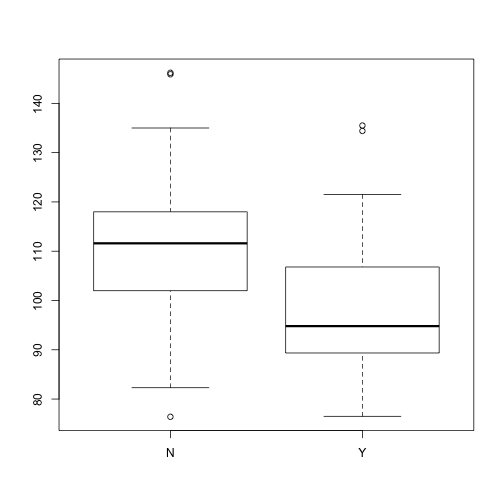This call to the 'boxplot' function has the format of “height = gall_YN” or “height is a function of gall.” This is the same format used for regression and other linear models in 'R'.

You can easily adjust the y-axis. Here is a boxplot of height inluding zero as the baseline. This is set using 'ylim ='. THe minimum comes first, then the max. Inorder to avoid having to hard-code in the maximum of the range I use the 'max' function within 'ylim'.

boxplot(height ~ gall_YN, ylim = c(0, max(height)))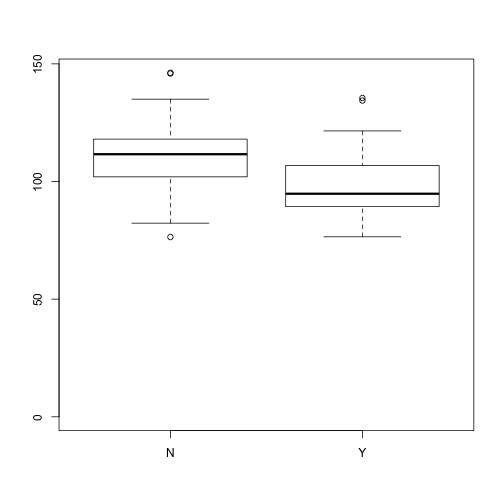Part III: Data analysis using paired t-tests

Stem Height analysis

Paired t.test

Divide data into two groups by creating an index.

i.N <- which(data\$gall_YN == "N")
i.Y <- which(data\$gall_YN == "Y")

Use these indices to calcualte means, SDs and SEs like before

data_height_means <- c(mean(height[i.N]), mean(height[i.Y]))
data_height_SD <- c(sd(height[i.N]), sd(height[i.Y]))
data_height_SE <- c(sd(height[i.N]), sd(height[i.Y]))/sqrt(n)

Plot the data using a barplot. There is a 'barplot' function in base 'R' but 'barplot2' in the 'gplots' library is more flexible and allows you to plot error bars.

library(gplots)

Create the barplot.

barplot2(data_height_means, ci.l = data_height_means - data_height_SE, ci.u = data_height_means +
data_height_SE, plot.ci = TRUE, ci.lty = 1, ci.color = 1, ci.lwd = 2, ci.width = 0.5,
col = c("grey", "green"), lwd = 2, width = 0.25, xlab = "Gall status", ylab = "Mean plant height (cm)",
names.arg = c("No Gall", "Gall"), axis.lty = 1, main = "Stem HEIGHT")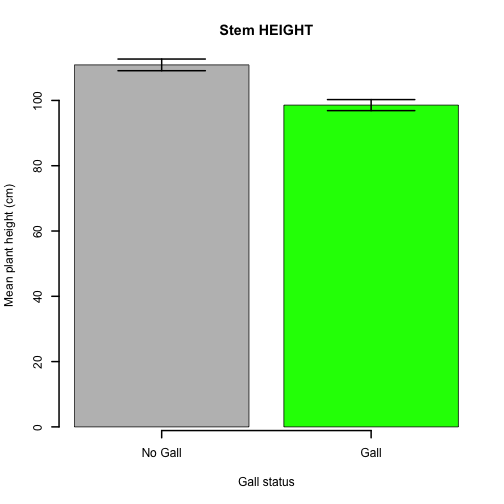Now, conduct a paired t-test. For the sake of completeness some of the above code is repeated. The t-test is run twice. Firt, the t-test is run using the 't.test' function and save to an 'R' object. The output is called up by typing the name of the object into the 'R' consol. Next, the t-test is run run directly so that the ouput is automatically displayed, but nothign is saved.

# Subsets
i.N <- which(data\$gall_YN == "N")
i.Y <- which(data\$gall_YN == "Y")

# Run paired t-test and save to an object
height.t.test <- t.test(height[i.N], height[i.Y], paired = TRUE)

# Run paired t-test directly
t.test(height[i.N], height[i.Y], paired = FALSE, var.equal = TRUE)
##
##  Two Sample t-test
##
## data:  height[i.N] and height[i.Y]
## t = 3.543, df = 68, p-value = 0.0007205
## alternative hypothesis: true difference in means is not equal to 0
## 95 percent confidence interval:
##   5.383 19.268
## sample estimates:
## mean of x mean of y
##    110.89     98.57

Its useful to save the t.test to an object so you can access the t-stat and p-value. This can be useful if you want to build plots that include this information within the plot.

# Look at the structure of the saved t-test of the object
str(height.t.test)
## List of 9
##  \$ statistic  : Named num 4.98
##   ..- attr(*, "names")= chr "t"
##  \$ parameter  : Named num 34
##   ..- attr(*, "names")= chr "df"
##  \$ p.value    : num 1.82e-05
##  \$ conf.int   : atomic [1:2] 7.3 17.4
##   ..- attr(*, "conf.level")= num 0.95
##  \$ estimate   : Named num 12.3
##   ..- attr(*, "names")= chr "mean of the differences"
##  \$ null.value : Named num 0
##   ..- attr(*, "names")= chr "difference in means"
##  \$ alternative: chr "two.sided"
##  \$ method     : chr "Paired t-test"
##  \$ data.name  : chr "height[i.N] and height[i.Y]"
##  - attr(*, "class")= chr "htest"

# Acessing just the t-statitic
height.t.test\$statistic
##    t
## 4.98

# Acessing and rounding the p-value
round(height.t.test\$p.value, 7)
##  1.82e-05

Stem Mass analysis

Calculate and save the means, SD and SEs for the stem masses

data_weight_STEM_means <- c(mean(weight_STEM[i.N]), mean(weight_STEM[i.Y]))
data_weight_STEM_SD <- c(sd(weight_STEM[i.N]), sd(weight_STEM[i.Y]))
data_weight_STEM_SE <- c(sd(weight_STEM[i.N]), sd(weight_STEM[i.Y]))/sqrt(n)
barplot2(data_weight_STEM_means, ci.l = data_weight_STEM_means - data_weight_STEM_SE,
ci.u = data_weight_STEM_means + data_weight_STEM_SE, plot.ci = TRUE, ci.lty = 1,
ci.color = 1, ci.lwd = 2, ci.width = 0.5, col = c("grey", "green"), lwd = 2,
width = 0.25, xlab = "Gall status", ylab = "Mean plant mass (g)", ylim = c(0,
15), names.arg = c("No Gall", "Gall"), axis.lty = 1, main = "Stem MASS")

text(x = 0.135, y = 14, labels = "Error Bars = +/- SE")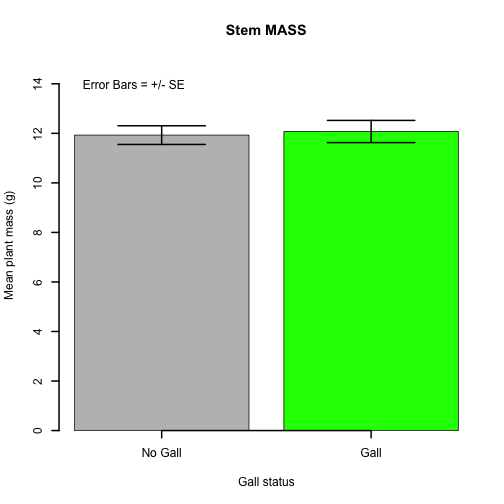T-test

# Correct - paired
weight.t.test <- t.test(weight_STEM[i.N], weight_STEM[i.Y], paired = TRUE)

# Wrong - not paired
t.test(weight_STEM[i.N], weight_STEM[i.Y], paired = FALSE, var.equal = TRUE)
##
##  Two Sample t-test
##
## data:  weight_STEM[i.N] and weight_STEM[i.Y]
## t = -0.176, df = 68, p-value = 0.8608
## alternative hypothesis: true difference in means is not equal to 0
## 95 percent confidence interval:
##  -1.798  1.506
## sample estimates:
## mean of x mean of y
##     11.93     12.07

Total mass of plant analysis

# stem + flower Correct - paired
t.test(weight_TOTAL[i.N], weight_TOTAL[i.Y], paired = TRUE)
##
##  Paired t-test
##
## data:  weight_TOTAL[i.N] and weight_TOTAL[i.Y]
## t = 1.167, df = 34, p-value = 0.2512
## alternative hypothesis: true difference in means is not equal to 0
## 95 percent confidence interval:
##  -0.5631  2.0831
## sample estimates:
## mean of the differences
##                    0.76

# Wrong - not paired
t.test(weight_TOTAL[i.N], weight_TOTAL[i.Y], paired = FALSE, var.equal = TRUE)
##
##  Two Sample t-test
##
## data:  weight_TOTAL[i.N] and weight_TOTAL[i.Y]
## t = 0.8036, df = 68, p-value = 0.4244
## alternative hypothesis: true difference in means is not equal to 0
## 95 percent confidence interval:
##  -1.127  2.647
## sample estimates:
## mean of x mean of y
##     13.35     12.59

FLOWER MASS VS 1: All plants

this plot creates some additional objects to make the plotting code cleaner and easier to recycle

data_weight_FLWR_means <- c(mean(weight_FLWR[i.N]), mean(weight_FLWR[i.Y]))
data_weight_FLWR_SD <- c(sd(weight_FLWR[i.N]), sd(weight_FLWR[i.Y]))
data_weight_FLWR_SE <- c(sd(weight_FLWR[i.N]), sd(weight_FLWR[i.Y]))/sqrt(n)
plot_means <- data_weight_FLWR_means
plot_SD <- data_weight_FLWR_SD
plot_SE <- data_weight_FLWR_SE
plot_CI.l <- plot_means - plot_SE
plot_CI.u <- plot_means + plot_SE
y_lab <- "Mean Flower Mass (g)"
y_lim <- c(0, 2)
main_title <- "Flower MASS"
barplot2(plot_means, ci.l = plot_CI.l, ci.u = plot_CI.u, plot.ci = TRUE, ci.lty = 1,
ci.color = 1, ci.lwd = 2, ci.width = 0.5, col = c("grey", "green"), lwd = 2,
width = 0.25, xlab = "Gall status", ylab = y_lab, ylim = y_lim, names.arg = c("No Gall",
"Gall"), axis.lty = 1, main = main_title)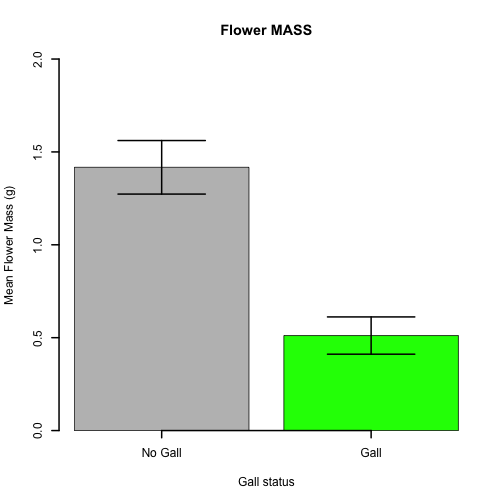text(x = 0.15, y = 1.85, labels = "Error Bars = +/- SE")
## Error: plot.new has not been called yet

T-test

weight_flwr.t.test <- t.test(weight_FLWR[i.N], weight_FLWR[i.Y], paired = TRUE)
t.test(weight_FLWR[i.N], weight_FLWR[i.Y], paired = FALSE, var.equal = TRUE)
##
##  Two Sample t-test
##
## data:  weight_FLWR[i.N] and weight_FLWR[i.Y]
## t = 3.647, df = 68, p-value = 0.0005147
## alternative hypothesis: true difference in means is not equal to 0
## 95 percent confidence interval:
##  0.4102 1.4013
## sample estimates:
## mean of x mean of y
##    1.4171    0.5114

Flower mass vs. 1: All plants

This code plots the average flower mass for galled and non-galled plants, and tests for a difference between the two groups using a paired t-test. In this case, we consider all plants; therefore, plants that didn't have a flower have a mass = 0. In the next section below, we'll consider only the mass of actualy flowers and drop all masses that are 0.

# Summary statistics for data
data_weight_FLWR_means <- c(mean(weight_FLWR[i.N]), mean(weight_FLWR[i.Y]))
data_weight_FLWR_SD <- c(sd(weight_FLWR[i.N]), sd(weight_FLWR[i.Y]))
data_weight_FLWR_SE <- c(sd(weight_FLWR[i.N]), sd(weight_FLWR[i.Y]))/sqrt(n)
# Save summary statistics to general R objects used for plotting.  These
# objects are recycled between plots so that the code can easily be
# re-purposed.
plot_means <- data_weight_FLWR_means
plot_SD <- data_weight_FLWR_SD
plot_SE <- data_weight_FLWR_SE

# Calculate confidence intervals
plot_CI.l <- plot_means - plot_SE
plot_CI.u <- plot_means + plot_SE

# Define axis labels
y_lab <- "Mean Flower Mass (g)"

# Define limits of y-axis
y_lim <- c(0, 2)

# Define title of plot
main_title <- "Flower mass"
# Create barplot
barplot2(plot_means, ci.l = plot_CI.l, ci.u = plot_CI.u, plot.ci = TRUE, ci.lty = 1,
ci.color = 1, ci.lwd = 2, ci.width = 0.5, col = c("grey", "green"), lwd = 2,
width = 0.25, xlab = "Gall status", ylab = y_lab, ylim = y_lim, names.arg = c("No Gall",
"Gall"), axis.lty = 1, main = main_title)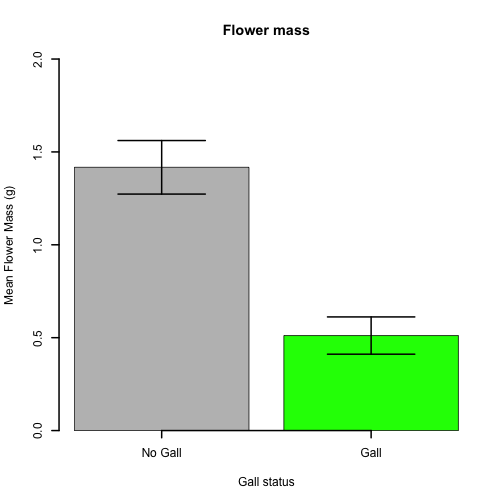An annotation can be added using the 'text' command.

text(x = 0.15, y = 1.85, labels = "Error Bars = +/- SE")
## Error: plot.new has not been called yet

Flower Mass vs 2: non-zero plants

In this code we consider the mass of flowers

working_data <- data\$weight_FLWR

which plants with mass > 0

i.FLWR_NOT_ZERO <- which(data\$weight_FLWR > 0)

Intersect between mass > 0 and galled

i.FLWR_NOT_ZERO.GALLED <- intersect(i.FLWR_NOT_ZERO, i.Y)

What pairs to these mass >0 and galled plants belong to?

pairs.FLWR_NOT_ZERO.GALLED <- data\$pair[i.FLWR_NOT_ZERO.GALLED]

Isolate galled plants in a pair where both have a flower

i.Y.2 <- which(data\$pair %in% pairs.FLWR_NOT_ZERO.GALLED & data\$gall_YN == "Y")

Isolate NON-galled plants in a pair where both have a flower

i.N.2 <- which(data\$pair %in% pairs.FLWR_NOT_ZERO.GALLED & data\$gall_YN == "N")

data_weight_FLWR_means <- c(mean(working_data[i.N.2]), mean(working_data[i.Y.2]))
data_weight_FLWR_SD <- c(sd(working_data[i.N.2]), sd(working_data[i.Y.2]))
data_weight_FLWR_SE <- c(sd(working_data[i.N.2]), sd(working_data[i.Y.2]))/sqrt(n)

plot_means <- data_weight_FLWR_means
plot_SD <- data_weight_FLWR_SD
plot_SE <- data_weight_FLWR_SE

plot_CI.l <- plot_means - plot_SE
plot_CI.u <- plot_means + plot_SE

y_lab <- "Mean Flower Mass (g)"
y_lim <- c(0, 2)

main_title <- "Flower MASS"

barplot2(plot_means, ci.l = plot_CI.l, ci.u = plot_CI.u, plot.ci = TRUE, ci.lty = 1,
ci.color = 1, ci.lwd = 2, ci.width = 0.5, col = c("grey", "green"), lwd = 2,
width = 0.25, xlab = "Gall status", ylab = y_lab, ylim = y_lim, names.arg = c("No Gall",
"Gall"), axis.lty = 1, main = main_title)

text(x = 0.15, y = 1.9, labels = "Error Bars = +/- SE")
text(x = 0.45, y = 1.5, labels = "n = 11")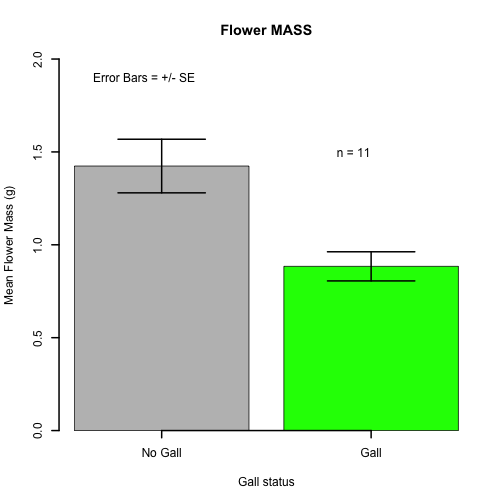Paired t-test to determine if there is a difference between the two groups.

weight_flwrno0.t.test <- t.test(weight_FLWR[i.N.2], weight_FLWR[i.Y.2], paired = TRUE)
t.test(weight_FLWR[i.N.2], weight_FLWR[i.Y.2], paired = FALSE, var.equal = TRUE)
##
##  Two Sample t-test
##
## data:  weight_FLWR[i.N.2] and weight_FLWR[i.Y.2]
## t = 1.965, df = 48, p-value = 0.05523
## alternative hypothesis: true difference in means is not equal to 0
## 95 percent confidence interval:
##  -0.01258  1.09258
## sample estimates:
## mean of x mean of y
##     1.424     0.884

Percent (%) of Mass in flower vs 1: all plants

percent_FLWR

# Create a copy of the flowering data
working_data <- data\$percent_FLWR

# Summary stats
data_means <- c(mean(working_data[i.N]), mean(working_data[i.Y]))
data_SD <- c(sd(working_data[i.N]), sd(working_data[i.Y]))
data_SE <- c(sd(working_data[i.N]), sd(working_data[i.Y]))/sqrt(n)

# Calculate confidence intervals
plot_CI.l <- data_means - data_SE
plot_CI.u <- data_means + data_SE

y_lab <- "Mean Portion Mass in Flower"
y_lim <- c(0, 0.15)

main_title <- "Portion mass in Flower"

barplot2(data_means, ci.l = plot_CI.l, ci.u = plot_CI.u, plot.ci = TRUE, ci.lty = 1,
ci.color = 1, ci.lwd = 2, ci.width = 0.5, col = c("grey", "green"), lwd = 2,
width = 0.25, xlab = "Gall status", ylab = y_lab, ylim = y_lim, names.arg = c("No Gall",
"Gall"), axis.lty = 1, main = main_title)

text(x = 0.125, y = 0.13, labels = "Error Bars = +/- SE")
text(x = 0.49, y = 0.13, labels = paste("N = ", length(i.Y), sep = ""))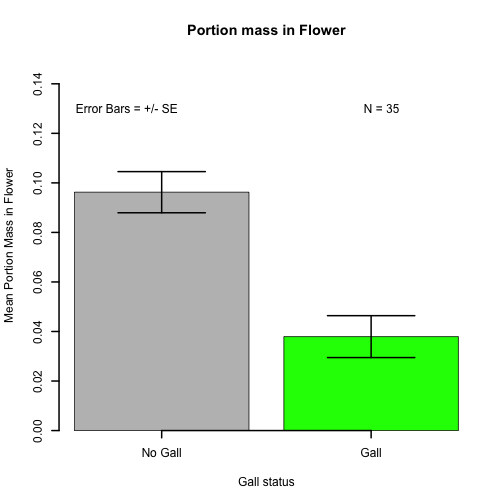t-test

percent_flwr.t.test <- t.test(percent_FLWR[i.N], percent_FLWR[i.Y], paired = TRUE)
t.test(percent_FLWR[i.N], percent_FLWR[i.Y], paired = FALSE, var.equal = TRUE)
##
##  Two Sample t-test
##
## data:  percent_FLWR[i.N] and percent_FLWR[i.Y]
## t = 3.478, df = 68, p-value = 0.0008867
## alternative hypothesis: true difference in means is not equal to 0
## 95 percent confidence interval:
##  0.02485 0.09177
## sample estimates:
## mean of x mean of y
##   0.09624   0.03793

VERSION 2: only flowering Data with mass > 0

working_data <- data\$percent_FLWR

which plants with mass > 0

i.FLWR_NOT_ZERO <- which(data\$percent_FLWR > 0)

Intersect between mass > 0 and galled

i.FLWR_NOT_ZERO.GALLED <- intersect(i.FLWR_NOT_ZERO, i.Y)

what pairs to these mass >0 and galled plants belong to?

pairs.FLWR_NOT_ZERO.GALLED <- data\$pair[i.FLWR_NOT_ZERO.GALLED]

Isolate galled plants in a pair where both have a flower

i.Y.2 <- which(data\$pair %in% pairs.FLWR_NOT_ZERO.GALLED & data\$gall_YN == "Y")

Isolate NON-galled plants in a pair where both have a flower

i.N.2 <- which(data\$pair %in% pairs.FLWR_NOT_ZERO.GALLED & data\$gall_YN == "N")

data_means <- c(mean(working_data[i.N.2]), mean(working_data[i.Y.2]))
data_SD <- c(sd(working_data[i.N.2]), sd(working_data[i.Y.2]))
data_SE <- c(sd(working_data[i.N.2]), sd(working_data[i.Y.2]))/sqrt(n)
plot_CI.l <- data_means - data_SE
plot_CI.u <- data_means + data_SE

y_lab <- "Mean Portion Mass in Flower"
y_lim <- c(0, 0.15)

main_title <- "Portion mass in Flower: non-zero"

barplot2(data_means, ci.l = plot_CI.l, ci.u = plot_CI.u, plot.ci = TRUE, ci.lty = 1,
ci.color = 1, ci.lwd = 2, ci.width = 0.5, col = c("grey", "green"), lwd = 2,
width = 0.25, xlab = "Gall status", ylab = y_lab, ylim = y_lim, names.arg = c("No Gall",
"Gall"), axis.lty = 1, main = main_title)

text(x = 0.125, y = 0.13, labels = "Error Bars = +/- SE")
text(x = 0.49, y = 0.13, labels = paste("N = ", length(i.Y.2), sep = ""))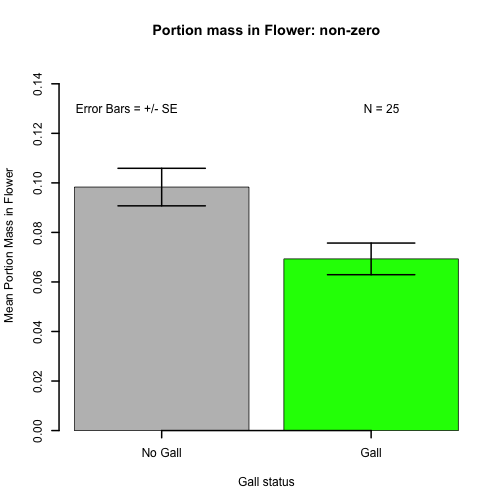T-test

percent_flwr.t.test <- t.test(percent_FLWR[i.N.2], percent_FLWR[i.Y.2], paired = TRUE)

t.test(percent_FLWR[i.N.2], percent_FLWR[i.Y.2], paired = FALSE, var.equal = TRUE)
##
##  Two Sample t-test
##
## data:  percent_FLWR[i.N.2] and percent_FLWR[i.Y.2]
## t = 1.748, df = 48, p-value = 0.08684
## alternative hypothesis: true difference in means is not equal to 0
## 95 percent confidence interval:
##  -0.004354  0.062338
## sample estimates:
## mean of x mean of y
##   0.09831   0.06932

Graph How many plants flowered?

table_out <- table(data\$gall_YN, data\$flower_F.NF)
## Error: all arguments must have the same length

prop_table_out <- prop.table(table_out, margin = 1)

Version 1

par(mfrow = c(1, 2))
pie(prop_table_out[1:2], labels = c("Flower", "Non-flower"), main = "Not Galled",
col = c("grey", 3))